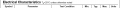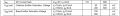# Transistor in Active and saturation region

#### mishra87

Joined Jan 17, 2016
1,000
Hi,

What is basic calculation for transistor to work in Active and saturation region. :
Lets take BC547 transistor in calculation :

Calculation with example :
Thanks

#### dl324

Joined Mar 30, 2015
15,838
What is basic calculation for transistor to work in Active and saturation region. :
Lets take BC547 transistor in calculation :

Calculation with example :
For BC547, Ib = Ic/20 for saturation mode operation.

For active mode operation, B-E junction forward biased, C-B junction reverse biased.

•cmartinez

#### mishra87

Joined Jan 17, 2016
1,000
For BC547, Ib = Ic/20 for saturation mode operation.

For active mode operation, B-E junction forward biased, C-B junction reverse biased.
I did not get still why does Ib = Ic/20 ???
Hfe = 110 - 800 for bc547

Could you explain a bit more about Active region.

#### bertus

Joined Apr 5, 2008
22,219
Hello,

We use the 1/10 to 1/20 of the collector current as base current to force the transistor in saturation.

Bertus

#### dl324

Joined Mar 30, 2015
15,838
I did not get still why does Ib = Ic/20 ???
Hfe = 110 - 800 for bc547
The datasheet assumes beta=20 for saturation mode:The 110-800 value is for VCE=5V and Ic=2mA. When you're operating in saturation mode, VCE is going to be 0.6V max.
Could you explain a bit more about Active region.
You just bias the transistor so that the junctions are biased as I indicated.

#### mishra87

Joined Jan 17, 2016
1,000
Examples :

Vcc = 5V
Vled = 1.6V
Vce(sat) = .25V
Ic= 20mA
hfe= 100
Vb = 3.3V

In saturation : Rc = (5-1.6-0.25)/20mA = 157.5 ohm
so ib= (20/100 ) = 200uA , lets say ib>200uA
Rb= (3.3-0.7)/200uA = 13K

Hope above calculation is correct for saturation region

Now : what is active region and how to do calculation for it.

#### mishra87

Joined Jan 17, 2016
1,000
As a novice , detailed explanation needed.

thanks

#### crutschow

Joined Mar 14, 2008
32,921
The active region is when the transistor is used as an AC or DC amplifier.
In this active region, where the collector-emitter voltage is more than a volt, the collector current equals the base current times the transistor current gain (β or Hfe from the data sheet, which can vary significantly from unit to unit).

The saturation region is when the transistor is used as an on-off switch.
In this saturation region, the base current is increased to 5-10% (forced Beta of 10 to 20) of the maximum collector current, so that the transistor is fully saturated, with a minimum collector-emitter voltage (turned completely on as a switch).
The other state is with no base current. where the transistor does not conduct (turned completely off as a switch).

Make sense?

•mishra87

#### bertus

Joined Apr 5, 2008
22,219
Hello,

Have a look at the attached PDF on the pages 2-15 and on.

Bertus

#### Attachments

• 2.4 MB Views: 14

#### yashnit

Joined Apr 23, 2019
1
Hi,

What is basic calculation for transistor to work in Active and saturation region. :
Lets take BC547 transistor in calculation :

Calculation with example :
Thanks
\
You can conclude the operation of a transistor if it is saturated or not by doing actual measurement. Monitor the collector-emitter voltage of your circuit with a DMM. If the reading is below 0.3V, the transistor is at saturation. Transistors are having saturation voltage range from 0.7V and below but for a circuit designed for hard saturation, the VCE will be lower. Figure below is the setup on how to measure the collector-emitter voltage of a transistor in circuit. Read More

#### dl324

Joined Mar 30, 2015
15,838
Examples :

Vcc = 5V
Vled = 1.6V
Vce(sat) = .25V
Ic= 20mA
hfe= 100
Vb = 3.3V

In saturation : Rc = (5-1.6-0.25)/20mA = 157.5 ohm
so ib= (20/100 ) = 200uA , lets say ib>200uA
Rb= (3.3-0.7)/200uA = 13K

Hope above calculation is correct for saturation region
Your calculations aren't correct. Try building the circuit to see if it works.

As I mentioned earlier, the datasheet indicates a beta of 20 for saturation mode. That changes the required base current.

$$\small I_B = I_C/20 = 1mA R_B = \frac{3.3V-0.7V}{1mA} = 2600\Omega$$

#### WBahn

Joined Mar 31, 2012
29,167
Examples :

Vcc = 5V
Vled = 1.6V
Vce(sat) = .25V
Ic= 20mA
hfe= 100
Vb = 3.3V

In saturation : Rc = (5-1.6-0.25)/20mA = 157.5 ohm
so ib= (20/100 ) = 200uA , lets say ib>200uA
Rb= (3.3-0.7)/200uA = 13K

Hope above calculation is correct for saturation region

Now : what is active region and how to do calculation for it.
This is meaningless without the circuit it applies to. Don't make us guess -- engineering is not about guessing.

•mishra87

#### mishra87

Joined Jan 17, 2016
1,000
This is meaningless without the circuit it applies to. Don't make us guess -- engineering is not about guessing.
Hey WBahn,

I am not guessing anything.
I have done calculation for a practical circuit and then i was asking my doubts through forum.
I hope analog people can understand my intentions.

Regards,

#### mishra87

Joined Jan 17, 2016
1,000
The active region is when the transistor is used as an AC or DC amplifier.
In this active region, where the collector-emitter voltage is more than a volt, the collector current equals the base current times the transistor current gain (β or Hfe from the data sheet, which can vary significantly from unit to unit).

The saturation region is when the transistor is used as an on-off switch.
In this saturation region, the base current is increased to 5-10% (forced Beta of 10 to 20) of the maximum collector current, so that the transistor is fully saturated, with a minimum collector-emitter voltage (turned completely on as a switch).
The other state is with no base current. where the transistor does not conduct (turned completely off as a switch).

Make sense?
Thanks !!!

#### mishra87

Joined Jan 17, 2016
1,000
\
You can conclude the operation of a transistor if it is saturated or not by doing actual measurement. Monitor the collector-emitter voltage of your circuit with a DMM. If the reading is below 0.3V, the transistor is at saturation. Transistors are having saturation voltage range from 0.7V and below but for a circuit designed for hard saturation, the VCE will be lower. Figure below is the setup on how to measure the collector-emitter voltage of a transistor in circuit. Read More
Marketed well to your website. !!!

#### WBahn

Joined Mar 31, 2012
29,167
Hey WBahn,

I am not guessing anything.
I have done calculation for a practical circuit and then i was asking my doubts through forum.
I hope analog people can understand my intentions.

Regards,
You aren't guessing because you know what the schematic is for the circuit you are talking about.

You are forcing US to guess because you won't share schematic with us. So we don't know whether you have a base resistor, or an emitter resistor, or a collector resistor, or what the emitter supply voltage is. We have to guess at all of that stuff. Why do you want to force the people that you are trying to get to help you to guess at what the circuit is that you are working with?

#### mishra87

Joined Jan 17, 2016
1,000
Further to discussion ..please find attched schematic and Vce waveform...

Why transistor not in saturation....vce is around 1.85 V